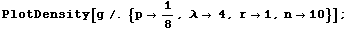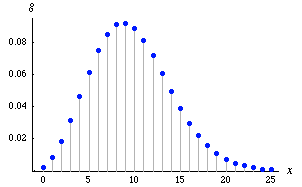### Order Statistics

Example 1: Continuous     Logistic distribution

Let random variable X have a Logistic distribution with pdf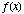:

In:=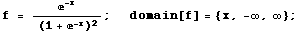Letdenote a sample of size n drawn on X, and let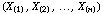denote the ordered sample, so that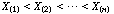. The pdf of the r th order statistic,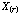, is given by the mathStatica function:

In:=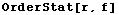Out=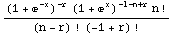The following diagram plots the pdf of the r th order statistic, as r increases from 1 to 10, given a sample of size n = 10: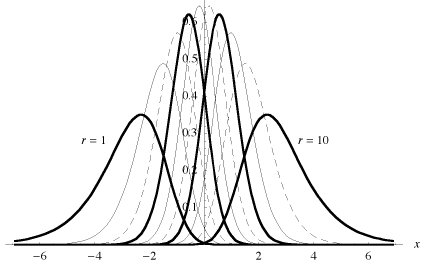The joint pdf ofand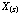, for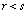, is given by:

In:=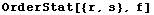Out:=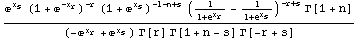A new feature in mathStatica 1.2 is that the OrderStat function now also supports discrete random variables. To illustrate, let random variable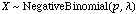with pmf:

In:=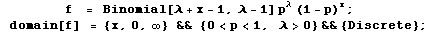Let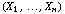denote a random sample of size n drawn on X, and let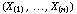denote the order statistics.

Then, the pmf of the r th order statistic,, denoted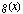, is given immediately by:

In:=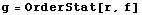Out:=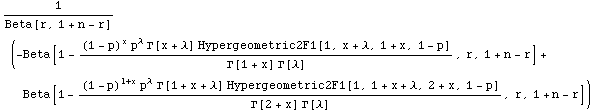with domain of support:

In:=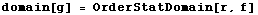Out:=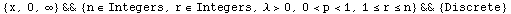The following diagram plots the pmf of the r th order statistic that we have just derived, when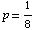,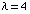and r = 1 and the sample size is n = 10:

In:=